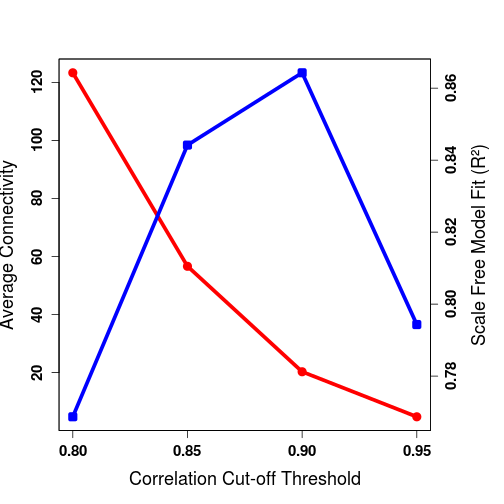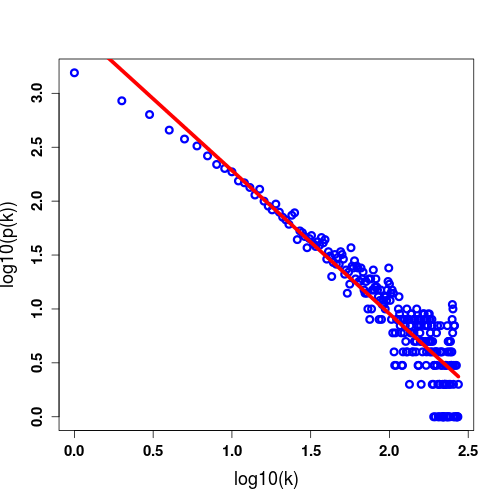ChlamyNET, a gene co-expression network for Chlamydomonas reinhardtii

ChlamyNET is a gene co-expression network for Chlamydomonas reinhardtti constructed using RNA-seq data. ChlamyNET aims at becoming an enabling technology for researchers studying the Chlamydomonas transcriptome.

The protocol for the analysis of raw sequencing data is based on the software tools Tophat and Cufflinks. The output of this protocol is then analyzed using the R package cummeRbund.

``````library("cummeRbund")
``````
``````## Loading required package: BiocGenerics
##
## Attaching package: 'BiocGenerics'
##
## The following objects are masked from 'package:parallel':
##
##     clusterApply, clusterApplyLB, clusterCall, clusterEvalQ,
##     clusterExport, clusterMap, parApply, parCapply, parLapply,
##     parLapplyLB, parRapply, parSapply, parSapplyLB
##
## The following object is masked from 'package:stats':
##
##     xtabs
##
## The following objects are masked from 'package:base':
##
##     anyDuplicated, append, as.data.frame, as.vector, cbind,
##     colnames, do.call, duplicated, eval, evalq, Filter, Find, get,
##     intersect, is.unsorted, lapply, Map, mapply, match, mget,
##     order, paste, pmax, pmax.int, pmin, pmin.int, Position, rank,
##     rbind, Reduce, rep.int, rownames, sapply, setdiff, sort,
##     table, tapply, union, unique, unlist, unsplit
##
##
## Attaching package: 'fastcluster'
##
## The following object is masked from 'package:stats':
##
##     hclust
##
##
## Attaching package: 'cummeRbund'
##
## The following object is masked from 'package:GenomicRanges':
##
##     promoters
##
## The following object is masked from 'package:IRanges':
##
##     promoters
##
## The following object is masked from 'package:BiocGenerics':
##
##     conditions
``````

The output of the analysis protocol based on Tophat and Cufflinks is stored in the file chlamyData.db that needs to be loaded using readCufflinks:

``````setwd("~/Documents/ChlamyNET_data")
data
``````
``````## CuffSet instance with:
##   20 samples
##   16624 genes
##   20736 isoforms
##   18061 TSS
##   18898 CDS
##   3158560 promoters
##   3431590 splicing
##   3158560 relCDS
``````

Selection of Differentially Expressed Genes

The selection of differentially expressed genes was performed using the standard methodology applied to the analysis of RNA-seq data. The logarithm of the gene expression levels measured in FPKM were computed and the delta method was used to estimate the variance of the log odds. Those genes that exhibited an adjusted p-value for the multiple testing lower than 0.05 were considered to be differentially expressed.

The information related to differentially expressed genes (DEGs) can be extracted using the functions genes and diffData:

``````gene.diff <- diffData(genes(data))
``````
``````##       gene_id        sample_1         sample_2 status   value_1   value_2
## 1 XLOC_000001 Fe_repleted_Min Fe_deficient_Min     OK  2.857180  4.444380
## 2 XLOC_000002 Fe_repleted_Min Fe_deficient_Min NOTEST  0.707877  0.464713
## 3 XLOC_000003 Fe_repleted_Min Fe_deficient_Min     OK  1.365800  1.831230
## 4 XLOC_000004 Fe_repleted_Min Fe_deficient_Min     OK  1.395880  1.328790
## 5 XLOC_000005 Fe_repleted_Min Fe_deficient_Min     OK  8.662940  7.652160
## 6 XLOC_000006 Fe_repleted_Min Fe_deficient_Min     OK 57.353400 39.839000
##   log2_fold_change test_stat   p_value  q_value significant
## 1        0.6373900 -1.321300 0.1864020 0.276998          no
## 2       -0.6071580  0.894250 0.3711880 1.000000          no
## 3        0.4230680 -1.220790 0.2221640 0.319614          no
## 4       -0.0710628  0.218149 0.8273130 0.878806          no
## 5       -0.1789900  0.589376 0.5556090 0.657194          no
## 6       -0.5256990  1.731700 0.0833275 0.141793          no
``````

We extract using the function subset the DEGs from each experiment.

DEGs in the Fe experiment:

``````sig.diff.Fe.replete.Min.Fe.deficient.Min <- subset(gene.diff,(sample_1 == "Fe_repleted_Min" & sample_2 == "Fe_deficient_Min" & significant == "yes"))
DEGs.Fe.replete.Min.Fe.deficient.Min <- sig.diff.Fe.replete.Min.Fe.deficient.Min[["gene_id"]]
length(DEGs.Fe.replete.Min.Fe.deficient.Min)
``````
``````##  181
``````
``````sig.diff.Fe.replete.Min.Fe.limited.Min <- subset(gene.diff,(sample_1 == "Fe_repleted_Min" & sample_2 == "Fe_limited_Min" & significant == "yes"))
DEGs.Fe.replete.Min.Fe.limited.Min <- sig.diff.Fe.replete.Min.Fe.limited.Min[["gene_id"]]
length(DEGs.Fe.replete.Min.Fe.limited.Min)
``````
``````##  1785
``````
``````sig.diff.Fe.deficient.Min.Fe.limited.Min <- subset(gene.diff,(sample_1 == "Fe_deficient_Min" & sample_2 == "Fe_limited_Min" & significant == "yes"))
DEGs.Fe.deficient.Min.Fe.limited.Min <- sig.diff.Fe.deficient.Min.Fe.limited.Min[["gene_id"]]
length(DEGs.Fe.deficient.Min.Fe.limited.Min)
``````
``````##  893
``````

DEGs in the H2O2 experiment:

``````sig.diff.H2O2t0.H2O2t05 <- subset(gene.diff,(sample_1 == "H2O2_t0" & sample_2 == "H2O2_t05" & significant == "yes"))
DEGs.H2O2t0.H2O2t05 <- sig.diff.H2O2t0.H2O2t05[["gene_id"]]
length(DEGs.H2O2t0.H2O2t05)
``````
``````##  3726
``````
``````sig.diff.H2O2t0.H2O2t1 <- subset(gene.diff,(sample_1 == "H2O2_t0" & sample_2 == "H2O2_t1" & significant == "yes"))
DEGs.H2O2t0.H2O2t1 <- sig.diff.H2O2t0.H2O2t1[["gene_id"]]
length(DEGs.H2O2t0.H2O2t1)
``````
``````##  6331
``````
``````sig.diff.H2O2t05.H2O2t1 <- subset(gene.diff,(sample_1 == "H2O2_t05" & sample_2 == "H2O2_t1" & significant == "yes"))
DEGs.H2O2t05.H2O2t1 <- sig.diff.H2O2t05.H2O2t1[["gene_id"]]
length(DEGs.H2O2t05.H2O2t1)
``````
``````##  3507
``````

DEGs in the O2 experiment:

``````sig.diff.4A.sor1 <- subset(gene.diff,(sample_1 == "singlet_4A" & sample_2 == "singlet_sor1" & significant == "yes"))
DEGs.4A.sor1 <- sig.diff.4A.sor1[["gene_id"]]
length(DEGs.4A.sor1)
``````
``````##  5087
``````

DEGs in the TAP experiment:

``````sig.diff.TAP.recipes <- subset(gene.diff,(sample_1 == "TAP_recipe1" & sample_2 == "TAP_recipe2" & significant == "yes"))
DEGs.TAP.recipes <- sig.diff.TAP.recipes[["gene_id"]]
length(DEGs.TAP.recipes)
``````
``````##  58
``````

DEGs in the Cu experiment:

``````sig.diff.Cu.deficient.Cu.sufficient <- subset(gene.diff,(sample_1 == "Cu_deficient" & sample_2 == "Cu_sufficient" & significant == "yes"))
DEGs.Cu.deficient.Cu.sufficient <- sig.diff.Cu.deficient.Cu.sufficient[["gene_id"]]
length(DEGs.Cu.deficient.Cu.sufficient)
``````
``````##  346
``````
``````sig.diff.Cu.deficient.Cu.deficient.crr1 <- subset(gene.diff,(sample_1 == "Cu_deficient" & sample_2 == "Cu_deficient_crr1" & significant == "yes"))
DEGs.Cu.deficient.Cu.deficient.crr1 <- sig.diff.Cu.deficient.Cu.deficient.crr1[["gene_id"]]
length(DEGs.Cu.deficient.Cu.deficient.crr1)
``````
``````##  5112
``````
``````sig.diff.Cu.deficient.Cu.deficient.crr12 <- subset(gene.diff,(sample_1 == "Cu_deficient" & sample_2 == "Cu_deficient_crr12" & significant == "yes"))
DEGs.Cu.deficient.Cu.deficient.crr12 <- sig.diff.Cu.deficient.Cu.deficient.crr12[["gene_id"]]
length(DEGs.Cu.deficient.Cu.deficient.crr12)
``````
``````##  4195
``````
``````sig.diff.Cu.deficient.crr1.Cu.deficient.crr12 <- subset(gene.diff,(sample_1 == "Cu_deficient_crr12" & sample_2 == "Cu_deficient_crr1" & significant == "yes"))
DEGs.Cu.deficient.crr1.Cu.deficient.crr12 <- sig.diff.Cu.deficient.crr1.Cu.deficient.crr12[["gene_id"]]
length(DEGs.Cu.deficient.crr1.Cu.deficient.crr12)
``````
``````##  6504
``````

DEGs in the N experiment:

``````sig.diff.N.repleted.N.deprived <- subset(gene.diff,(sample_1 == "N_repleted" & sample_2 == "N_deprived" & significant == "yes"))
DEGs.N.repleted.N.deprived <- sig.diff.N.repleted.N.deprived[["gene_id"]]
length(DEGs.N.repleted.N.deprived)
``````
``````##  4006
``````

DEGs in the S experiment:

``````sig.diff.S.repleted.S.deprived <- subset(gene.diff,(sample_1 == "S_repleted" & sample_2 == "S_deprived" & significant == "yes"))
DEGs.S.repleted.S.deprived <- sig.diff.S.repleted.S.deprived[["gene_id"]]
length(DEGs.S.repleted.S.deprived)
``````
``````##  5527
``````
``````sig.diff.S.repleted.S.deprived.ars11 <- subset(gene.diff,(sample_1 == "S_repleted_ars11" & sample_2 == "S_deprived_ars11" & significant == "yes"))
DEGs.S.repleted.S.deprived.ars11 <- sig.diff.S.repleted.S.deprived.ars11[["gene_id"]]
length(DEGs.S.repleted.S.deprived.ars11)
``````
``````##  7060
``````
``````sig.diff.S.repleted.S.repleted.ars11 <- subset(gene.diff,(sample_1 == "S_repleted" & sample_2 == "S_repleted_ars11" & significant == "yes"))
DEGs.S.repleted.S.repleted.ars11 <- sig.diff.S.repleted.S.repleted.ars11[["gene_id"]]
length(DEGs.S.repleted.S.repleted.ars11)
``````
``````##  3090
``````
``````sig.diff.S.deprived.S.deprived.ars11 <- subset(gene.diff,(sample_1 == "S_deprived" & sample_2 == "S_deprived_ars11" & significant == "yes"))
DEGs.S.deprived.S.deprived.ars11 <- sig.diff.S.deprived.S.deprived.ars11[["gene_id"]]
length(DEGs.S.deprived.S.deprived.ars11)
``````
``````##  7227
``````

The final set of DEGs is obtained by collecting the DEGs from each experiment and removing duplicates using the function unique:

``````DEGs <- c(DEGs.Fe.replete.Min.Fe.deficient.Min,DEGs.Fe.replete.Min.Fe.limited.Min,DEGs.Fe.deficient.Min.Fe.limited.Min,DEGs.H2O2t0.H2O2t05,DEGs.H2O2t0.H2O2t1,DEGs.H2O2t05.H2O2t1,DEGs.4A.sor1,DEGs.TAP.recipes,DEGs.Cu.deficient.Cu.sufficient,DEGs.Cu.deficient.Cu.deficient.crr1,DEGs.Cu.deficient.Cu.deficient.crr12,DEGs.Cu.deficient.crr1.Cu.deficient.crr12,DEGs.N.repleted.N.deprived,DEGs.S.repleted.S.deprived,DEGs.S.repleted.S.deprived.ars11,DEGs.S.repleted.S.repleted.ars11,DEGs.S.deprived.S.deprived.ars11)

DEGs <- unique(DEGs)

length(DEGs)
``````
``````##  13699
``````

The overall number of DEGs that are considered for the construction of ChlamyNET is 13699.

In order to free memory, unnecessary variables are removed and garbage is collected using the functions rm and gc:

``````rm(sig.diff.Fe.replete.Min.Fe.deficient.Min)
rm(sig.diff.Fe.replete.Min.Fe.limited.Min)
rm(sig.diff.Fe.deficient.Min.Fe.limited.Min)
rm(sig.diff.H2O2t0.H2O2t05)
rm(sig.diff.H2O2t0.H2O2t1)
rm(sig.diff.H2O2t05.H2O2t1)
rm(sig.diff.4A.sor1)
rm(sig.diff.TAP.recipes)
rm(sig.diff.Cu.deficient.Cu.sufficient)
rm(sig.diff.Cu.deficient.Cu.deficient.crr1)
rm(sig.diff.Cu.deficient.Cu.deficient.crr12)
rm(sig.diff.Cu.deficient.crr1.Cu.deficient.crr12)
rm(sig.diff.N.repleted.N.deprived)
rm(sig.diff.S.repleted.S.deprived)
rm(sig.diff.S.repleted.S.deprived.ars11)
rm(sig.diff.S.repleted.S.repleted.ars11)
rm(sig.diff.S.deprived.S.deprived.ars11)
rm(DEGs.Fe.replete.Min.Fe.deficient.Min)
rm(DEGs.Fe.replete.Min.Fe.limited.Min)
rm(DEGs.Fe.deficient.Min.Fe.limited.Min)
rm(DEGs.H2O2t0.H2O2t05)
rm(DEGs.H2O2t0.H2O2t1)
rm(DEGs.H2O2t05.H2O2t1)
rm(DEGs.4A.sor1)
rm(DEGs.TAP.recipes)
rm(DEGs.Cu.deficient.Cu.sufficient)
rm(DEGs.Cu.deficient.Cu.deficient.crr1)
rm(DEGs.Cu.deficient.Cu.deficient.crr12)
rm(DEGs.Cu.deficient.crr1.Cu.deficient.crr12)
rm(DEGs.N.repleted.N.deprived)
rm(DEGs.S.repleted.S.deprived)
rm(DEGs.S.repleted.S.deprived.ars11)
rm(DEGs.S.repleted.S.repleted.ars11)
rm(DEGs.S.deprived.S.deprived.ars11)
rm(gene.diff)
gc()
``````
``````##           used  (Mb) gc trigger  (Mb) max used  (Mb)
## Ncells 3624422 193.6    5543382 296.1  4467397 238.6
## Vcells 2708432  20.7   48898844 373.1 60285450 460.0
``````

Selection of a correlation threshold to determine co-expression

Once the DEGs have been selected it is necesseary to establish a criterion to determine when two genes are co-expressed. Our criterion is going to be based on the correlation between gene expression profiles or expression levels.

The functions annotation and fpkm are used to extract the relevant information about gene annotation and expression levels or expression profiles:

``````gene.features <- annotation(genes(data))
gene.fpkm <- fpkm(genes(data))
``````
``````##       gene_id  sample_name     fpkm  conf_hi   conf_lo quant_status
## 1 XLOC_000001 Cu_deficient 27.82120 35.56970 20.072700           OK
## 2 XLOC_000002 Cu_deficient  2.19048  3.22123  1.159720           OK
## 3 XLOC_000003 Cu_deficient  2.39916  3.19103  1.607290           OK
## 4 XLOC_000004 Cu_deficient  1.30034  1.76393  0.836753           OK
## 5 XLOC_000005 Cu_deficient 27.25370 33.80730 20.700100           OK
## 6 XLOC_000006 Cu_deficient 25.40310 31.64860 19.157700           OK
##      stdev
## 1 3.874250
## 2 0.515375
## 3 0.395935
## 4 0.231795
## 5 3.276800
## 6 3.122750
``````

Next, we store the expression levels measured in FPKM (gene expression profiles) of the DEGs selected in the previous step and stored them in a matrix:

``````matrix.levels <- matrix(nrow=20,ncol=length(DEGs))

for(i in 1:length(DEGs))
{
matrix.levels[,i] <- subset(gene.fpkm,(gene_id == DEGs[i]))[["fpkm"]]
}
gc()
``````
``````##           used  (Mb) gc trigger  (Mb) max used  (Mb)
## Ncells 3660138 195.5    5543382 296.1  4467397 238.6
## Vcells 5484892  41.9   16023172 122.3 60285450 460.0
``````

Naming the columns of matrix.levels:

``````setwd("~/Documents/ChlamyNET_data")
cre.names <- as.vector(cre.names[["V1"]])
colnames(matrix.levels) <- cre.names
gc()
``````
``````##           used  (Mb) gc trigger  (Mb) max used  (Mb)
## Ncells 3664686 195.8    5543382 296.1  4467397 238.6
## Vcells 5507128  42.1   16023172 122.3 60285450 460.0
``````

We use the absolute value of the Pearson correlation coefficient between gene expression profiles across the different conditions to determine the level of co-expression between the selected genes.

``````abs.gene.correlation <- abs(cor(matrix.levels))
rm(matrix.levels)
gc()
``````
``````##             used   (Mb) gc trigger   (Mb)  max used   (Mb)
## Ncells   3664636  195.8    5543382  296.1   4467397  238.6
## Vcells 192922911 1471.9  213306791 1627.5 193240487 1474.4
``````

In order to decide which correlation value is high enough to consider that two genes are significantly co-expressed we used a criterion that establishes a compromise between the generation of a scale-free network and a high network density. Most biological networks characterized so far are scale-free which makes this property the most common metric for the rational selection of a gene correlation threshold. A range of correlation thresholds were considered. For each possible correlation cut-off value we determined how close the corresponding network was to fullfil the scale-free condition by computing the R2 of the linear regression for the logarithmic transform of the node degree distribution. Additionaly, for each possible cut-off value we used the average node degree as a meassurement of the network density.

``````## Considered correlation values
thresholds <- seq(from=0.80, by=0.05, to=0.95)
thresholds
``````
``````##  0.80 0.85 0.90 0.95
``````
``````## For each correlation value the mean connectivity and scale free R2 are stored
mean.connectivities <- vector(length=4,mode="numeric")
scale.free.R2 <- vector(length=4,mode="numeric")

for(i in 1:length(thresholds))
{
## Vector to store the degree or connectivity of each gene
connectivity <- vector(length=nrow(abs.gene.correlation),mode="numeric")

for(j in 1:nrow(abs.gene.correlation))
{
## The connectivity of a gene is computed as the number of genes that exhibit a
## correlation value over the analyzed threshold
connectivity[j] <- sum(abs.gene.correlation[j,] > thresholds[i]) - 1
}

## The mean connectivity is stored in the corresponding vector
mean.connectivities[i] <- mean(connectivity)

## The node distribution is computed using table
degree.distribution <- table(connectivity)
## Disconected nodes (nodes with degree 0) are discarded
degree.distribution <- degree.distribution[-1]
## The names of degree.distribution represent the possible degrees
degrees <- as.numeric(names(degree.distribution))
## Linear regression is used to check the adjustment to scale free
lm.r <- lm(log10(degree.distribution) ~ log10(degrees))
s.lm <- summary(lm.r)

## The R2 for the scale free property is stored in the corresponding vector
}

mean.connectivities
``````
``````##  123.304475  56.635083  20.296226   4.780495
``````
``````scale.free.R2
``````
``````##  0.7687083 0.8441823 0.8642736 0.7943325
``````

The next graph shows the mean connectivity and R2 for each correlation threshold.

``````par(mar=c(5,4,4,5)+.1)
plot(thresholds,mean.connectivities,type="o",col="red",lwd=5,ylab="",xlab="",cex.axis=1.25,font.axis=2)
mtext(side=1,"Correlation Cut-off Threshold",cex=1.5,line=3)
mtext(side=2,"Average Connectivity",cex=1.5,line=3)
par(new=TRUE)
plot(thresholds,scale.free.R2,type="o",col="blue",xaxt="n",yaxt="n",xlab="",ylab="",lwd=5,pch=0,cex.axis=1.25,font.axis=2)
axis(4,font=2,cex.axis=1.25)
mtext("Scale Free Model Fit (R²)",side=4,line=3,cex=1.5)
``````We observed that for increasing correlation thresholds the density of the network decreases whereas the fit to the scale-free property increases until the cut-off value is too restrictive and the network starts to deteriorate. Indeed, the scale-free model fit exhibits a maximum at a correlation value of 0.90 with an R2 equal to 0.86. For this threshold each gene has an average of approximately 20 neighbours which corresponds to a high enough network density. According to this we chose an absolute Pearson correlation threshold of 0.90 to consider that two genes are significantly co-expressed.

``````cor.threshold <- 0.90

## Vector to store the degree or connectivity of each gene
connectivity <- vector(length=nrow(abs.gene.correlation),mode="numeric")

for(j in 1:nrow(abs.gene.correlation))
{
## The connectivity of a gene is computed as the number of genes that exhibit a
## correlation value over the analyzed threshold
connectivity[j] <- sum(abs.gene.correlation[j,] > cor.threshold) - 1
}

## The node distribution is computed using table
degree.distribution <- table(connectivity)
## Disconected nodes (nodes with degree 0) are discarded
degree.distribution <- degree.distribution[-1]
## The names of degree.distribution represent the possible degrees
degrees <- as.numeric(names(degree.distribution))
## Linear regression is used to check the adjustment to scale free
lm.r <- lm(log10(degree.distribution) ~ log10(degrees))
s.lm <- summary(lm.r)

m <- s.lm[["coefficients"]][2,1]
n <- s.lm[["coefficients"]][1,1]

plot(log10(degrees),log10(degree.distribution),pch=1,cex=1.25,lwd=3,col="blue",xlab="log10(k)",ylab="log10(p(k))",cex.lab=1.5,cex.axis=1.25,font.axis=2)
lines(log10(degrees),m*log10(degrees)+n,col="red",lwd=5)
``````